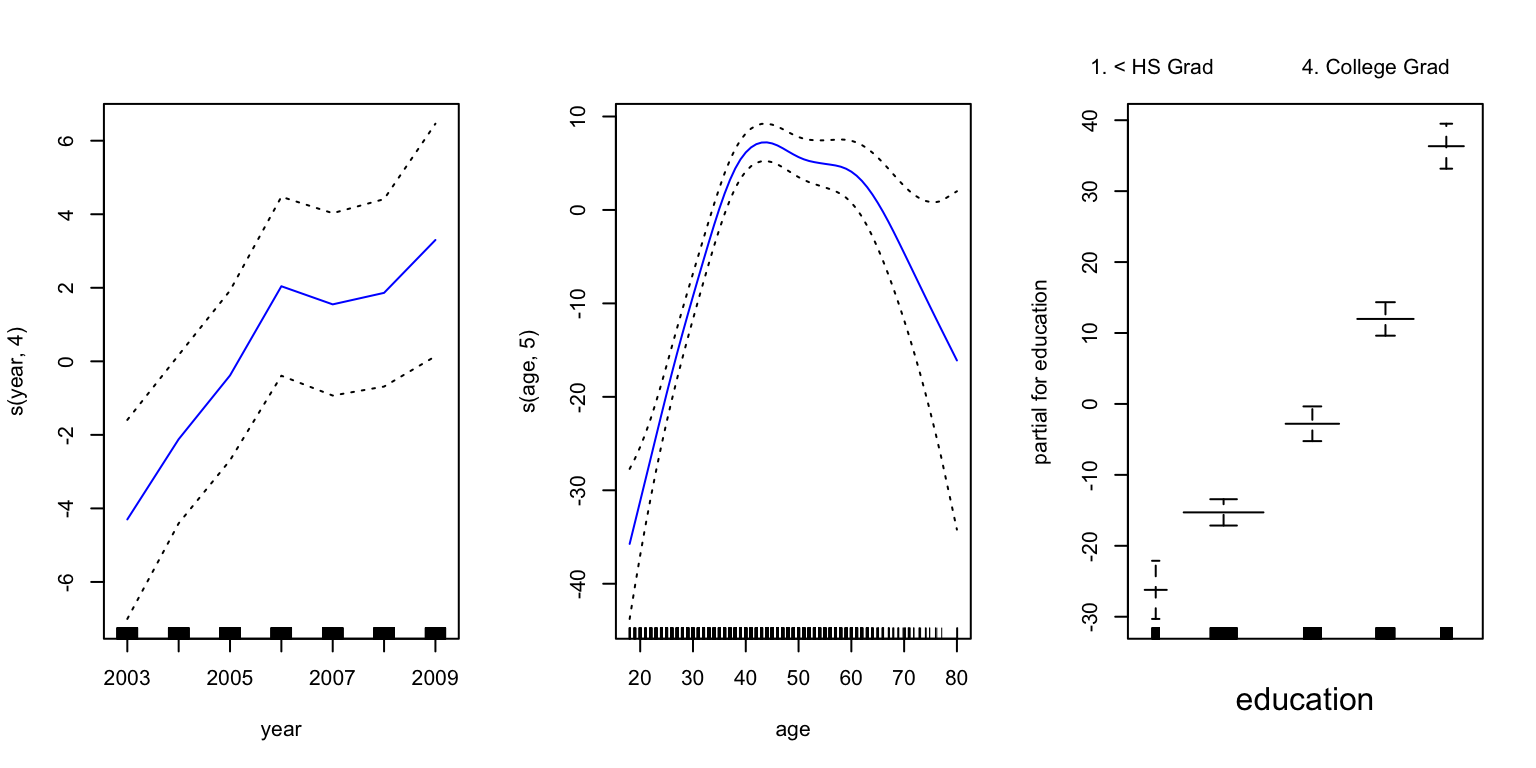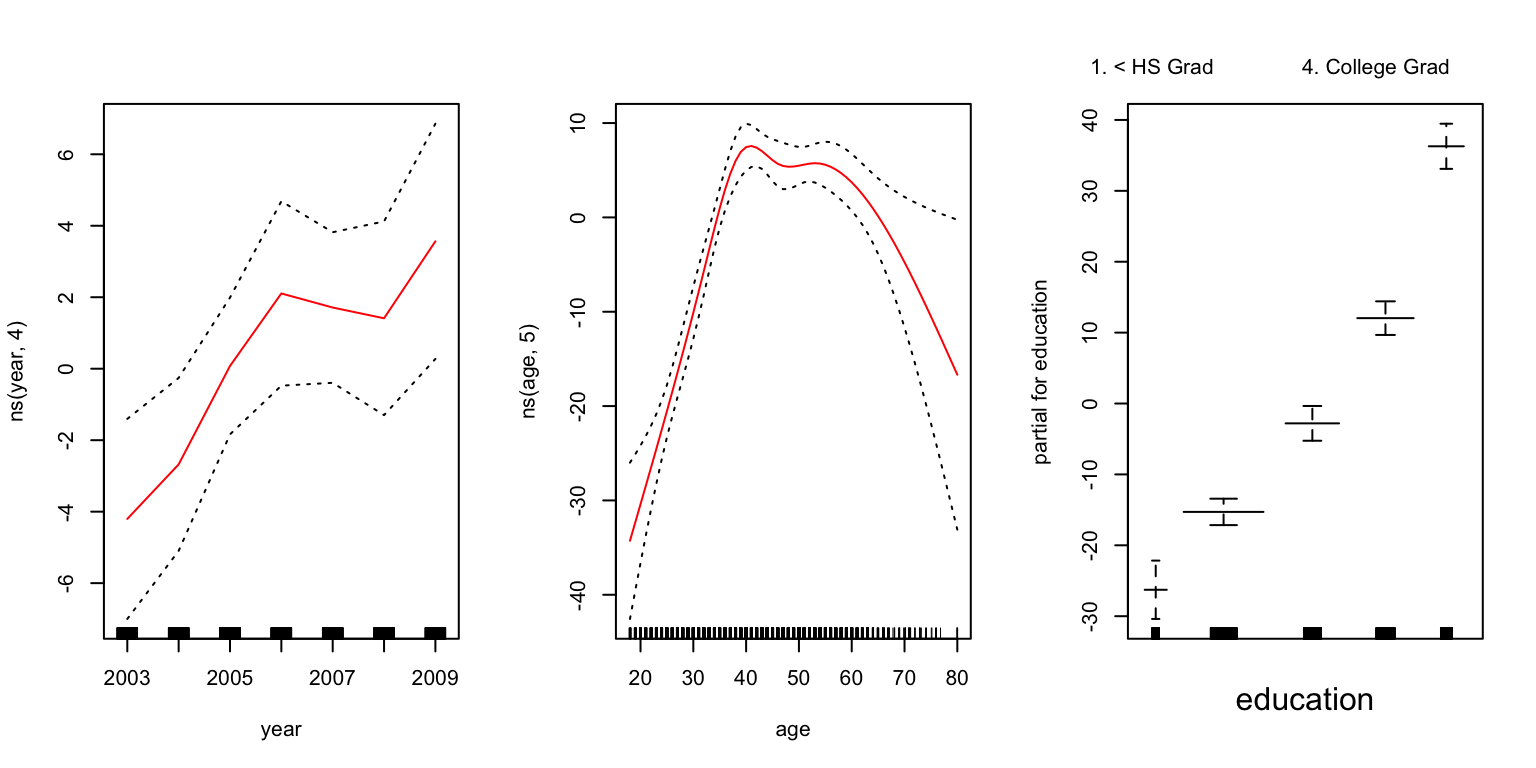``````library(ggplot2) # graphics library
library(MASS)    # contains data sets
library(ISLR)    # contains code and data from the textbook
library(knitr)   # contains kable() function
library(boot)    # contains cross-validation functions
library(gam)     # needed for additive models``````
``## Loading required package: splines``
``## Loading required package: foreach``
``## Loaded gam 1.14-4``
``options(scipen = 4)  # Suppresses scientific notation``

### 1. Changing the author field and file name.

##### (b) Rename this file to “Lab02_YourHameHere.Rmd”, where YourNameHere is changed to your own name.

The next portion of the lab gets you to carry out the Lab in §5.3 of ISLR (Pages 191 - 193). You will want to have the textbook Lab open in front you as you go through these exercises. The ISLR Lab provides much more context and explanation for what you’re doing.

### 2. The Validtion Set Approach

You will need the `Auto` data set from the `ISLR` library in order to complete this exercise.

Please run all of the code indicated in §5.3.1 of ISLR, even if I don’t explicitly ask you to do so in this document.

##### Use `qplot` to construct a scatterplot of `mpg` vs `horsepower`. Use `stat_smooth()` to overlay a linear, quadratic, and cubic polynomial fit to the data.
``# Edit me``
##### (b) Use the command `set.seed(1)` to set the seed of the random number generator. This will ensure that your answers match those in the text.
``# Edit me``
##### (c) Use the `sample()` command to construct `train`, a vector of observation indexes to be used for the purpose of training your model.
``# Edit me``
##### (c) Fit a linear model regression `mpg` on `horsepower`, specifying `subset = train`. Save this in a variable called `lm.fit`.
``# Edit me``
##### (d) Calculate the MSE of `lm.fit` on the test set (i.e., all points that are not in `train`)
``# Edit me``
##### (e) Use the `poly()` command to fit a quadratic regression model of `mpg` on `horsepower`, specifying `subset = train`. Save this in a variable called `lm.fit2`.
``# Edit me``
##### Is the coefficient of the quadratic term statistically significant?
``# Edit me``
##### Calculate the test MSE of `lm.fit2`. How does it compare to the linear regression fit?
``# Edit me``
##### (f) Use the `poly()` command to fit a cubic regression model of `mpg` on `horsepower`, specifying `subset = train`. Save this in a variable called `lm.fit3`.
``# Edit me``
##### Is the coefficient of the cubic term statistically significant?
``# Edit me``
##### Calculate the test error of the cubic fit.
``# Edit me``
##### (g) How do the test errors of the three models compare? Which of the three models should we use?
``# Edit me``

### 3. Leave-One-Out Cross-Validation

This exercise introduces you to the `cv.glm()` command from the `boot` library, which automates K-fold cross-validation for Generalized Linear Models (GLMs). Linear regression is one example of a GLM. Logistic regression is another. GLMs are not the same as Generalized Additive Models (GAMs).

Please run all of the code indicated in §5.3.2 of ISLR, even if I don’t explicitly ask you to do so in this document.

##### (a) Use the `glm` command to fit a linear regression of `mpg` on `horsepower`. Call the resulting model `glm.fit` Confirm that this gives the same coefficient estimates as a linear model fit with the `lm` command.

Note: You should fit the model to the entire data, not just to the training data.

``# Edit me``
##### (b) Run all of the code in §5.3.2. Construct a plot of `cv.error`, the vector of LOOCV error estimates for polynomials of degree 1-5.

Note: The computations take some time to run, so I’ve set `cache = TRUE` in the code chunk header to make sure that the code below doesn’t re-execute at knit time unless this particular chunk has changed.

``# Edit me (insert cross-validation code here)``
``# Edit me (insert plot code here)``
##### (c) Which degree model has the lowest LOOCV estimate of test error?
``# Edit me``

### 4. K-fold Cross-validation

Please run all of the code indicated in §5.3.3 of ISLR

##### (a) Run all of the code in the \(k\)-Fold Cross-validation Lab section.
``# Edit me``
##### (b) Construct a plot of 10-fold CV error vs. degree.
``# Edit me``
##### (c) Which model has the lowest 10-fold CV estimate of test error?
``# Edit me``

### 5. Additive Models and Splines

Please run all of the code indicated in §7.8.3 of ISLR, up until the loading of the `akima` package. We have not yet studied logistic regression, so you are not asked to do the logistic regression analysis that starts at the bottom of p. 297. The material on ANOVA testing may also be unfamiliar to you. You may skip it.

##### (a) Think carefully about what each line of code below is doing. Write comments in the code below to explain what each line is responsible for.
``````# Your comment here
gam1 <- lm(wage ~ ns(year, 4) + ns(age, 5) + education, data=Wage)

gam.m3 <- gam(wage ~ s(year, 4) + s(age, 5) + education, data=Wage)

par(mfrow=c(1,3))

plot(gam.m3, se=TRUE,col="blue")````````````# Your comment here
plot.gam(gam1, se=TRUE, col="red")````````````# Your comment here
gam.m1 <- gam(wage ~ s(age,5) + education, data=Wage)

gam.m2 <- gam(wage ~ year + s(age, 5) + education, data=Wage)

anova(gam.m1, gam.m2, gam.m3, test="F")``````
``````## Analysis of Deviance Table
##
## Model 1: wage ~ s(age, 5) + education
## Model 2: wage ~ year + s(age, 5) + education
## Model 3: wage ~ s(year, 4) + s(age, 5) + education
##   Resid. Df Resid. Dev Df Deviance       F    Pr(>F)
## 1      2990    3711731
## 2      2989    3693842  1  17889.2 14.4771 0.0001447 ***
## 3      2986    3689770  3   4071.1  1.0982 0.3485661
## ---
## Signif. codes:  0 '***' 0.001 '**' 0.01 '*' 0.05 '.' 0.1 ' ' 1``````
``````# Your comment here
summary(gam.m3)``````
``````##
## Call: gam(formula = wage ~ s(year, 4) + s(age, 5) + education, data = Wage)
## Deviance Residuals:
##     Min      1Q  Median      3Q     Max
## -119.43  -19.70   -3.33   14.17  213.48
##
## (Dispersion Parameter for gaussian family taken to be 1235.69)
##
##     Null Deviance: 5222086 on 2999 degrees of freedom
## Residual Deviance: 3689770 on 2986 degrees of freedom
## AIC: 29887.75
##
## Number of Local Scoring Iterations: 2
##
## Anova for Parametric Effects
##              Df  Sum Sq Mean Sq F value      Pr(>F)
## s(year, 4)    1   27162   27162  21.981 0.000002877 ***
## s(age, 5)     1  195338  195338 158.081   < 2.2e-16 ***
## education     4 1069726  267432 216.423   < 2.2e-16 ***
## Residuals  2986 3689770    1236
## ---
## Signif. codes:  0 '***' 0.001 '**' 0.01 '*' 0.05 '.' 0.1 ' ' 1
##
## Anova for Nonparametric Effects
##             Npar Df Npar F  Pr(F)
## (Intercept)
## s(year, 4)        3  1.086 0.3537
## s(age, 5)         4 32.380 <2e-16 ***
## education
## ---
## Signif. codes:  0 '***' 0.001 '**' 0.01 '*' 0.05 '.' 0.1 ' ' 1``````
``````# Your comment here
preds <- predict(gam.m2, newdata=Wage)